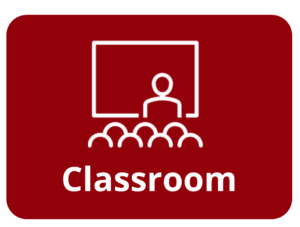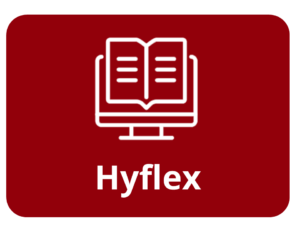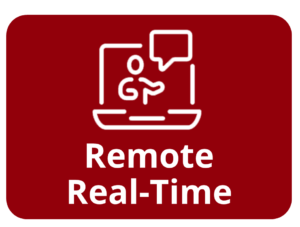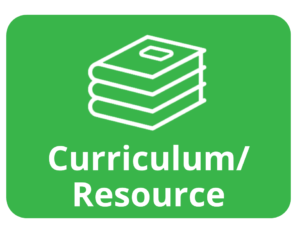Subject:  Math
Skills:  Numbers and Operations, Algebra and Algebraic Thinking, Geometry
Class Type:  Classroom, Remote real-time, Hyflex
Lesson Format:  Print, Online
NRS Level(s):  ABE 4
Math Standards:  Numbers and Operations, Algebra and Algebraic Thinking, Geometry, Math Practices 1, Math Practices 2, Math Practices 3, Math Practices 4, Math Practices 5, Math Practices 7